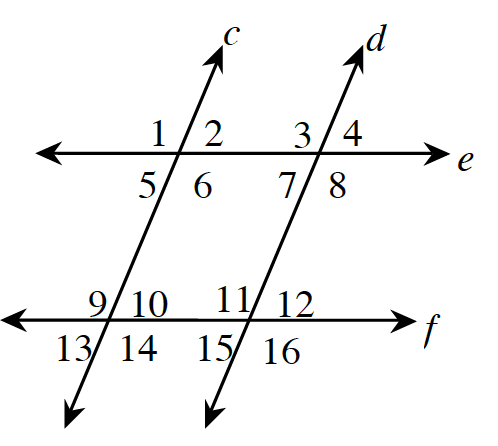### Home > CC3 > Chapter Ch9 > Lesson 9.1.3 > Problem9-33

9-33.

Based on the given information, determine which pairs of lines, if any, are parallel. If none are necessarily parallel, write “none.”

Alternate interior angles and corresponding angles are equal only if the lines cut by the transversal are parallel.
Conversely, if the angles are equal the lines must be parallel.

1. $m∠2 = m∠7$

These are alternate interior angles.

2. $m∠3 = m∠11$

These are corresponding angles.

$e$ and $f$

3. $m∠1 = m∠12$

In the diagram below, angles 1 and 12 are equal.
Do any two lines look parallel?

4. $m∠13 = m∠12$

Vertical angles are always equal.

$c$ and $d$

5. $∠6$ and $∠7$ are supplementary.

Angle 6 would be supplementary to angle 7 if angle 7 was equal to angle 2.